### Time Reversal

Bryan W. Roberts | LSE

Quantum Investigations
A conference in honour of Miklós Rédei

Why does time reversal matter?

The arrow of time
(Kaon decay)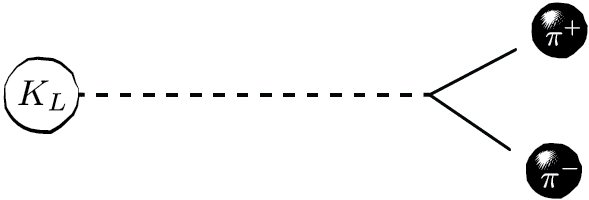Statistics
(Superselection)

$$\phi^{fer}+\phi^{bos} = mixed$$

Nature of matter
(Kramers degeneracy)

time-symmetric fermions
Fermions: $$[T,H]=0 \Rightarrow H$$ is degenerate
$$\Rightarrow$$ more magnetically susceptible

Animal RightsWhat would it even mean to 'reverse' time?

Standard picture of time reversal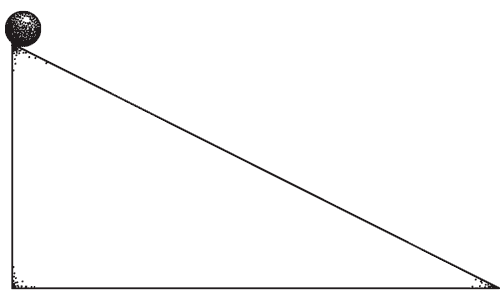Imagine a 'film' of a process, and then reverse it.

Standard meaning of time reversal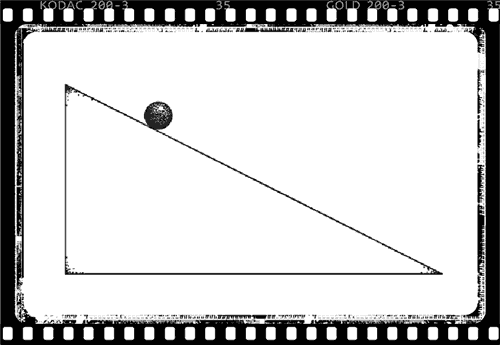$$t \mapsto -t$$
$$(Q,P) \mapsto (Q,-P)$$
$$\sigma_z \mapsto -\sigma_z$$
$$(E,B)\mapsto (E,-B)$$
$$\psi \mapsto T\psi$$

Physics Skeptics: Does $$T$$ deserve the name?

"'reversal of the direction of motion' is perhaps a more felicitous, though longer, expression than 'time inversion'"

Wigner (1931)

Physics Skeptics: Does $$T$$ deserve the name?

"This is a difficult topic for the novice, partly because the term time reversal is a misnomer; it reminds us of science fiction. Actually what we do in this section can be more appropriately characterized by the term reversal of motion."

Sakurai (1994)

Physics Skeptics: Does $$T$$ deserve the name?

"the term 'time reversal' is misleading, and the operation . . . would be more accurately described as motion reversal."

Ballentine (1998)

Philosopher Skeptics: Does $$T$$ deserve the name?

"the books identify precisely that transformation as the transformation of 'time-reversal.' ... The thing is that this identification is wrong. ... [Time reversal] can involve nothing whatsoever other than reversing the velocities of the particles

Albert (2000)

Philosopher Skeptics: Does $$T$$ deserve the name?

"David Albert . . . argues—rightly in my opinion—that the traditional definition of [time-reversal invariance], which I have just given, is in fact gibberish. It does not make sense to time-reverse a truly instantaneous state of a system"

Callender (2000)

Philosopher Skeptics: Does $$T$$ deserve the name?

"time reversal should leave the states intrinsically untouched and just change their order. ... If we cleave to that understanding of time reversal, none of the counterexamples Roberts offers constitutes a failure of [Curie's Principle]"

Castellani and Ismael (2016)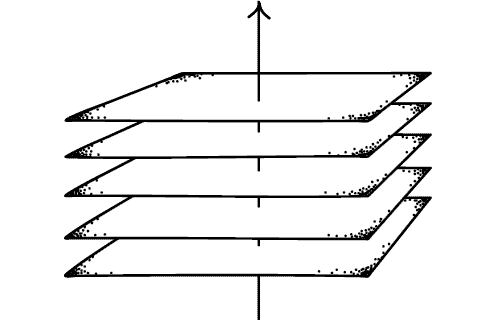Albert-Callender 'Pancake' Account:
Things 'just lie there' like an inert pancake

Time reversal can change the order of the pancakes, but not transform them

Today's Thesis:
Against the Pancake account, the standard meaning of time reversal (really! no need for 'motion reversal') can be derived

...from reasonable assumptions about the nature of time.

"Three Myths About Time Reversal" (BWR 2017)
philsci-archive.pitt.edu/12305/

Problems with the Pancake Account

Or, why things don't 'just lie there'

Observation 1.Temporally oriented properties are not meaningless.

Observation 2.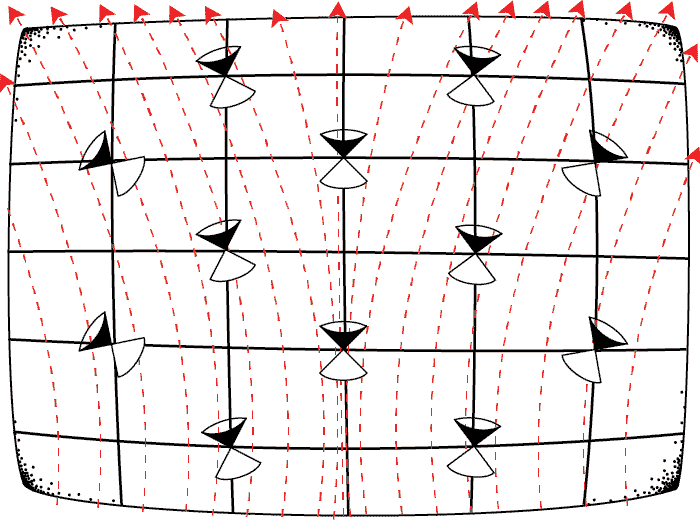Temporally oriented properties can be modelled mathematically.
Let $$\tau^a$$ be a temporal orientation

• Toy Field: $$\xi^a := c_1\tau^a + c_2$$
• Serious Field: $$F_{ab}$$ such that $$F^a(\xi^b,q) = qF^a_{\;b}\xi^b$$

($$F^a$$ is a mapping from a future-directed $$\xi^a$$ and charge $$q$$ to a vector)

Observation 3.

A harmonic oscillator is manifestly time reversal invariant.

Oscillator phase-space diagram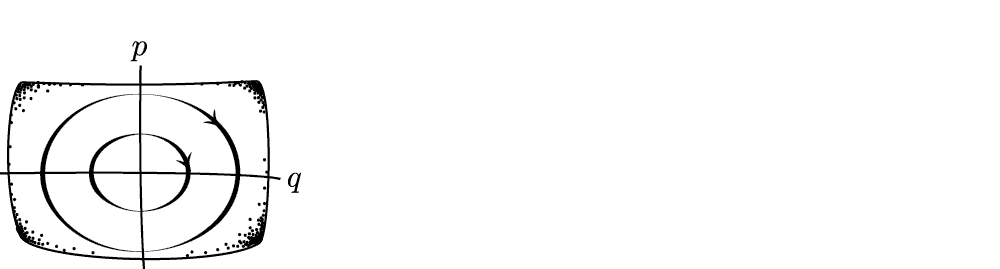Albert-Callender 'Pancake Reversal'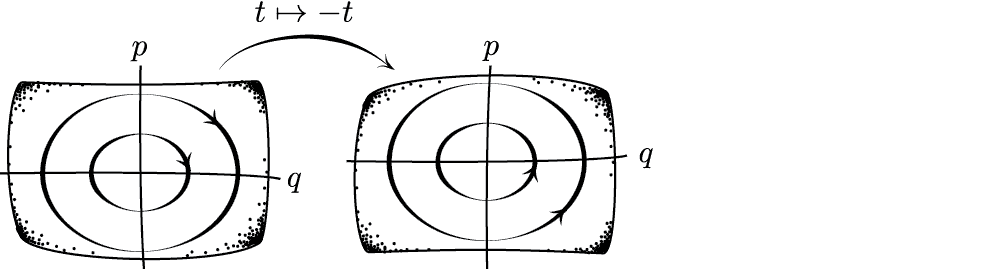Velocity and momentum in opposite directions!

Standard Time-Reversal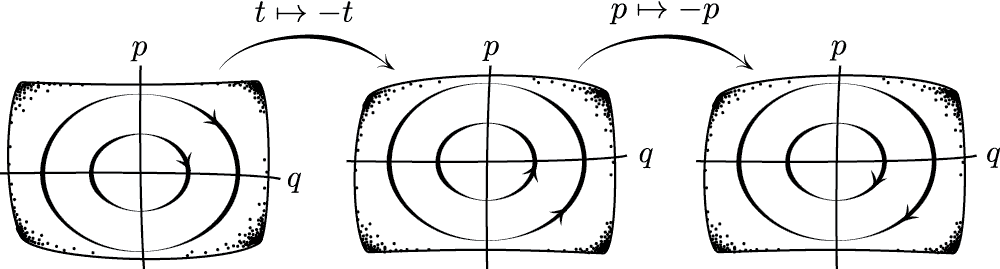Velocity and momentum in the same direction (BWR 2013)

### Two approaches

Temporal
Properties
of Matter

Temporal
Spacetime
Structure

#### A three stage approach

Time reversal satisfies:

1. Unitarity or Antiunitarity
2. Antiunitarity
3. Particular Transformation Properties

General Form of Time Reversal: $$\psi(t) \mapsto T\psi(-t)$$

Note that at this stage, $$T$$ could be the identity

0th stage: What even counts as order-reversal?

• Standard Account: $$t \mapsto -t$$
• Peterson Reversal (2015 SHPMP): $$t \mapsto \tfrac{1}{e^{t}}$$

Both satisfy order-reversal:
$$t>t' \Leftrightarrow f(t) < f(t')$$

0th stage: What even counts as order-reversal:

• (1) Order reversal: $$t < t' \Rightarrow f(t)>f(t')$$
• (2) No 'Stretching': $$t\mapsto f(t)$$ is linear
• (3) Involution: $$f(f(t))=t$$

Fact: (1)+(2)+(3) $$\Rightarrow$$ $$f(t) = -t+t_0$$ with $$t_0\in\mathbb{R}$$

Time-translation symmetry: can choose $$t_0=0 \Rightarrow f:t\mapsto -t$$

#### A three stage approach

Time reversal satisfies:

1. Unitarity or Antiunitarity
2. Antiunitarity
3. Particular Transformation Properties

Unitarity:
(1) $$U^*U=UU^*=I$$
(2) $$U(a\psi+b\phi)=aU\psi+bU\phi$$
$$\Leftrightarrow \langle U\psi,U\phi \rangle = \langle \psi,\phi \rangle$$

Antiunitarity:
(1) $$U^*U=UU^*=I$$
(2) $$U(a\psi+b\phi)=a^*U\psi+b^*U\phi$$
$$\Leftrightarrow \langle U\psi,U\phi \rangle = \langle \psi,\phi \rangle^*$$

Wigner Thm gloss: If $$T$$ preserves probabilities,

$|\langle T\psi,T\phi \rangle|^2 = |\langle \psi,\phi \rangle|^2,$

then $$T$$ is unitary or antiunitary.

Uhlhorn's Thm: If $$\mathrm{dim}\mathcal{H}>2$$ and $$\mathbf{T}$$ is 'orthogonality preserving',

$$\Psi\bot\Phi\;\;$$ iff $$\;\;\mathbf{T}\Psi\bot \mathbf{T}\Phi$$

then it is uniquely implemented by an operator $$T$$ that is unitary or antiunitary.

Uhlhorn's Thm gloss: If 'mutual impossibility' is independent of the direction of time, then $$T$$ is unitary or antiunitary.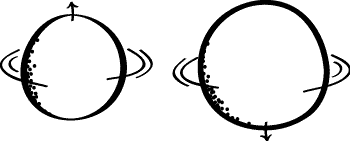$$T$$ can reasonably be assumed to satisfy the premise.

Philosophers agree so far.

Albert-Callender-Castellani-Ismael:
$$T=I$$ is the identity (and thus unitary)

#### A three stage approach

Time reversal satisfies:

1. Unitarity or Antiunitarity
2. Antiunitarity
3. Particular Transformation Properties

Argument 1.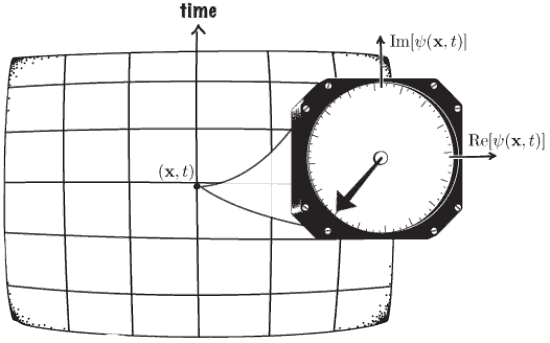Argument 2. Let $$TQT^{-1}$$ and $$TPT^{-1}=-P$$.

$$Ti\hbar T^{-1} = T[Q,P]T^{-1}$$
$$= [TQT^{-1},TPT^{-1}] = [Q,-P]$$
$$= -i\hbar$$

$$T$$ is not unitary $$\Rightarrow T$$ is antiunitary
by our previous discussion

Classical analogue: Given a Poisson manifold $$(M,\{\,,\,\})$$, time reversal is anticanonical.

$$T:C^\infty(M)\rightarrow C^\infty(M)$$ does not preserve the Poisson bracket. Rather: $\{Tp,Tq\}=\{-p,q\} = -\{p,q\}$

Another approach

Time reversal invariance (TRI)

An invariance of a dynamical theory maps possible trajectories to possible trajectories

Equivalent Statements about $$(\mathcal{H},t\mapsto e^{-itH})$$

• $$(\mathcal{H},t\mapsto e^{-itH})$$ is time reversal invariant
• If $$\psi(t)$$ is a solution, then $$T\psi(-t)$$ is too.
• $$Te^{itH}=e^{-itH}T$$

Argument 3.1 (Theorem). Let $$T:\mathcal{H}\rightarrow\mathcal{H}$$ be a (unitary or antiunitary) bijection. If there exists at least one self-adjoint $$H$$ (densely defined) satisfying,

1. (positive energy) $$\;0\leq \langle \psi,H\psi\rangle$$
2. (non-trivial) $$\;H\neq0$$
3. ($$T$$-reversal invariant) $$\;Te^{itH}=e^{-itH}T$$

Then $$T$$ is antiunitary.

Proof ⇊

Back Up ⇈

Proof. Condition iii) implies that $$e^{itH} = Te^{-itH}T^{-1} = e^{T(-itH)}T^{-1}$$, which implies that $$itH = -TitHT^{-1}$$. Now, suppose for reductio that $$T$$ is unitary and hence linear. Then we can conclude from the above that $$itH =-itTHT^{-1}$$, and hence $$THT=-H.$$ Since unitary operators preserve inner products, this gives $$\langle\psi,H\psi\rangle = \langle T\psi,TH\psi\rangle = -\langle T\psi,HT\psi\rangle.$$ But condition i) implies both $$\langle\psi,H\psi\rangle$$ and $$\langle T\psi,HT\psi\rangle$$ are nonnegative, so we have,

$0 \leq \langle\psi,H\psi\rangle = -\langle T\psi,HT\psi\rangle \leq 0.$

It follows that $$\langle\psi,H\psi\rangle = 0$$ for all $$\psi$$ in the domain of $$H$$. Since $$H$$ is densely defined, this is only possible if $$H$$ is the zero operator, contradicting condition ii. Therefore, since T is not unitary, it can only be antiunitary.

Argument 3.2 (Theorem). Let $$(M,\Omega)$$ be a symplectic manifold, and $$T$$ a symplectic or antisymplectic diffeo. If there exists at least one smooth function $$h:M\rightarrow\mathbb{R}$$ such that:

1. (energy) $$h$$ is bounded below but not above;
2. ($$T$$-reversal invariant) If $$c(t)$$ is an integral curve of the Hamiltonian vector field $$X_h$$, then so is $$\tau\circ c(-t)$$;

Then $$T$$ is antisymplectic.

Consequence of denying antiunitarity: Even an system with no interactions would fail to be time-reversal invariant.

Earman (2004): "the symptom of a perverse view"

#### A three stage approach

Time reversal satisfies:

1. Unitarity or Antiunitarity
2. Antiunitarity
3. Particular Transformation Properties

Question. Can we explain why:
$$Q\mapsto Q$$?
$$P\mapsto -P$$?
$$\sigma_z\mapsto -\sigma_z$$

Momentum generates spatial translations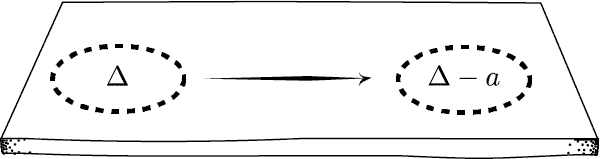$$e^{-iaP}(E_\Delta) e^{iaP}=E_{\Delta-a}$$

where $$Q=\int_{\mathbb{R}}\lambda dE_\lambda$$

Fact. Suppose the 'meaning' of time reversal does not depend on location in space:

$e^{-iaP}Te^{iaP}=T.$

Then time reversal maps $$P\mapsto-P$$

Proof: $$e^{-iaP}=Te^{-iaP}T^{-1}=e^{T(-iaP)T^{-1}}=e^{iaTPT^{-1}} \;\Rightarrow\; TPT^{-1}=-P$$

Uniqueness is established in BWR 2017 Proposition 2

Fact. Suppose the 'meaning' of time reversal does not depend on orientation in space:

$e^{-ia\sigma_z}Te^{ia\sigma_z}=T.$

Then time reversal maps $$\sigma_z\mapsto-\sigma_z$$

Uniqueness is established in BWR 2017 Proposition 3

Three places to get off the train:

1. Mutual impossibility independent of time's arrow
$$\Rightarrow T$$ is unitary or antiunitary
2. One non-trivial system is time symmetric
$$\Rightarrow T$$ is antiunitary
3. Homogeneity, isotropy, etc. of time-reversal
$$\Rightarrow$$ transformation rules for momentum, spin, etc.

Bonus Proposition!

$$P$$-invariance was replaced by $$CP$$, then $$CPT$$. Will we always have a replacement of the form $$UT$$?

Yes.

Proposition. For any quantum theory with a unitary dynamics $$t\mapsto e^{-itH}$$, there exists a unitary operator $$U$$ such that $$UT$$-invariance holds, where $$T$$ is the (antiunitary) time-reversal operator.

Proof ⇊

Back Up ⇈

Proposition. For any quantum theory $$(\mathcal{H},t\mapsto e^{-itH})$$, there exists a unitary operator $$U$$ such that $$UT$$-invariance holds, where $$T$$ is the (antiunitary) time-reversal operator.

Proof. Let $$T$$ be the (antiunitary) time reversal operator. Let $$K$$ be the (antiunitary) complex conjugation operator associated with $$H$$, and define $$U := KT^{-1}$$. Then $$U$$ is unitary, since it is the product of two antiunitary operators. Moreover, $$[K,H]=0$$, and so $$[UT,H]= [KT^{-1}T,H]=[K,H]=0$$, which implies that the theory is invariant under $$UT$$.

### Two approaches

Temporal
Properties
of Matter

Temporal
Spacetime
Structure

Malament (2004)

Temporal Properties of the EM-Field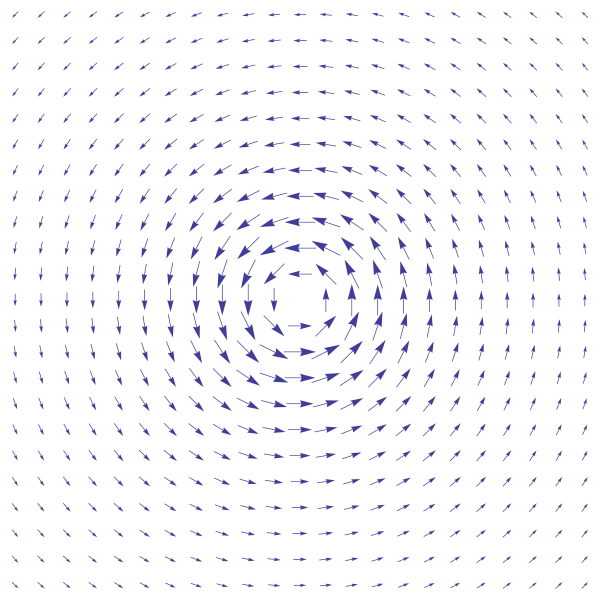"[$$B$$] can no more just lie there than an angular velocity vector field can"; instead, $$T:B\mapsto-B$$

Malament (2004)

Spacetime structures in the EM-FieldWith a time orientation $$\tau^a$$, let $$T:\tau^a\mapsto-\tau^a$$

Malament (2004)

Analyze the way $$\tau^a$$ 'hooks up' to physical quantities to induce the transformation rules for time reversal.

• $$T:F_{ab}\mapsto -F_{ab}$$
• $$T:J^a\mapsto -J^a$$
• $$T:E^a\mapsto E^a$$
• $$T:B^a\mapsto -B^a$$

A quantum analogue:

Theorem (Varadarajan). If $$\tau$$ is time reversal in the inhomogeneous Lorentz group, and if a representation $$\alpha$$ on $$\mathrm{Aut}(L)$$ of a Hilbert lattice $$L$$ satisfies,

• (a) (non-trivial) Rep of the translation subgroup is non-trivial; and
• (b) (positive energy) Spectrum is in the future light cone;

then $$T:=\alpha(\tau)$$ is antiunitary.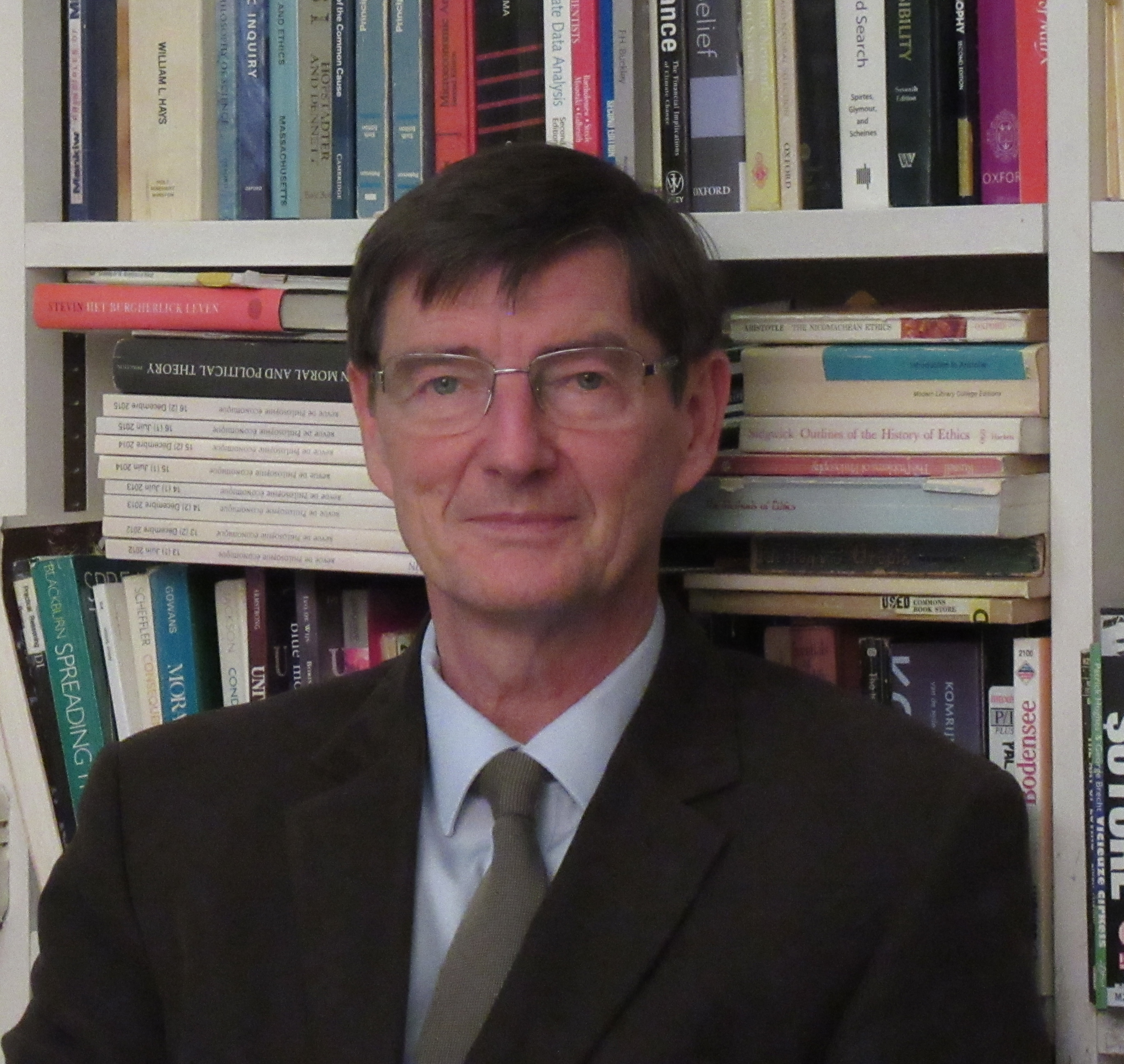### Open questions

• Generalising Varadarajan: Can this argument be generalised to more general nets of von Neumann algebras on a (temporally orientable) relativistic spacetime? ($$\rightarrow$$ Miklós)

### Open questions

• Lagrangian Framework: Can an argument analogous to the Hamiltonian approach to deriving time reversal be carried out in the context of Lagrangian mechanics?

### Open questions

• Classical transformation rules: Can the derivation of the quantum transformation rules for time reversal be carried over to classical mechanics?

#### finThese slides were written in html using reveal.js